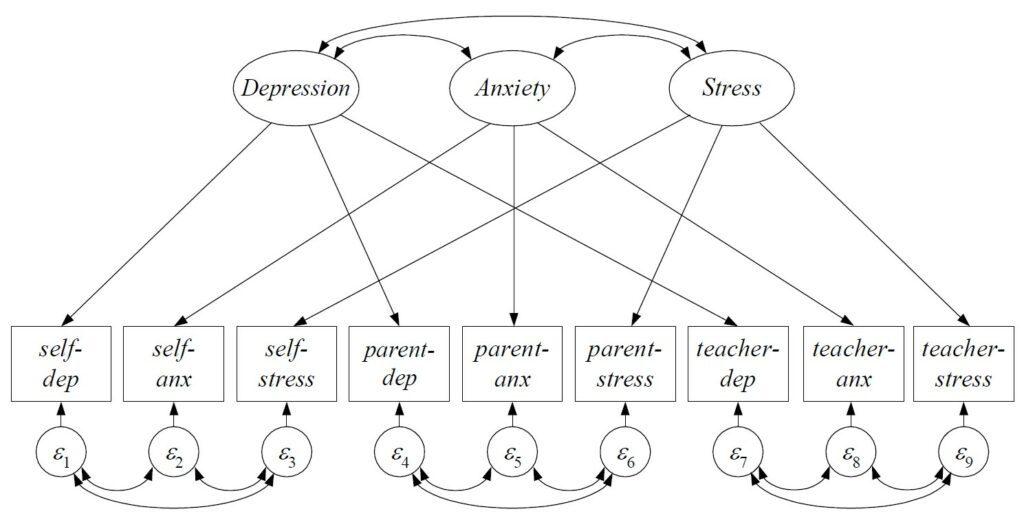# How do I know if my structural equation model fits the data well?This is one of the most common questions we receive and, unfortunately, there are no quick answers. However, there are some initial guidelines that can be followed when assessing the fit of an SEM. For most SEMs, the goal of the analysis is to define a model that results in predicted values of the summary statistics (sometimes called “moment structures” consisting of the variances, covariances, and means of the observed variables) that are as close as possible to the values that were observed in the sample. SEMs that result in a closer correspondence between the predicted and the observed summary statistics obtain a better fit compared to models that do not.

The most common measure of model fit is the chi-square statistic which tests the null hypothesis that the population variances, covariances, and means are precisely equal to those that are implied by the model. A significant chi-square test (say p<.05) leads to rejection of the null hypothesis and the conclusion that the model does not adequately reproduce the population summary statistics. Although widely used, the chi-square test is often over-powered and commonly leads to model rejection and thus a wide array of fit indices are available to augment the chi-square test. One class of indices are the relative fit indices that compare the fit of the model to some pre-defined baseline model; examples include the CFI, TLI, and IFI, and values exceeding .90 or .95 are often taken to indicate good fit. Another class of indices are the absolute fit indices that evaluate model fit in terms of model degrees-of-freedom; the most common example is the RMSEA and values falling below .05 are often taken to indicate good fit. Finally, examination of modification indices (sometimes called LaGrange multipliers or score tests) suggest restrictions that have been imposed on the model that do not correspond to the sample data, the freeing of which would improve overall model fit. There are many (many) other measures of fit and this is just a very brief summary of model evaluation in SEM. Further details can be found in the readings below.

Browne, M. W., & Cudeck, R. (1992). Alternative ways of assessing model fit. Sociological Methods & Research, 21, 230-258.

Hu, L. T., & Bentler, P. M. (1999). Cutoff criteria for fit indexes in covariance structure analysis: Conventional criteria versus new alternatives. Structural Equation Modeling: A Multidisciplinary Journal, 6, 1-55.

West, S. G., Taylor, A. B., & Wu, W. (2012). Model fit and model selection in structural equation modeling. In Handbook of Structural Equation Modeling (R. Hoyle, Ed.), 209-231.

## What’s the best way to determine the number of latent classes in a finite mixture analysis?

Selecting the number of classes (or components) is one of the most challenging decisions to make when fitting a finite mixture model (including latent class analysis and latent profile analysis). In this post, we talk through the conventional wisdom on class enumeration, as well as when this breaks down.

## What are ROC curves and how are these used to aid decision making?

ROC curves are used to identify cut points on continuous measures at which to make binary decisions. An example is a depression screener used to identify people who may qualify for a major depression diagnosis. This post explains the concepts behind ROC curves and how they are used for decision making.

## I fit a multilevel model and got the warning message “G Matrix is Non-Positive Definite.” What does this mean and what should I do about it?

Received the cryptic warning message “G matrix is non-positive definite”? Learn what this means and what to do about it.

## What exactly qualifies as intensive longitudinal data and why am I not able to use more traditional growth models to study stability and change over time?

This post considers the unique features of intensive longitudinal data (ILD) relative to other more traditional data structures and how we can appropriately analyze ILD given these features

## A reviewer recently asked me to comment on the issue of equivalent models in my structural equation model. What is the difference between alternative models and equivalent models within an SEM?

An equivalent model can be thought of as a re-parameterization of the original model. In other words, it is just a different way of “packaging” the same information in the data and no equivalent model can be distinguished from another based on fit alone. If you were to fit a series of equivalent models to the same sample data you obtain exactly the same chi-square test statistic, RMSEA, CFI, TLI, and any other omnibus measure of fit. It is often best to treat this as a limitation of any given study and to potentially present one or a small number of equivalent model options to the reader so that these too might be considered as plausible representations of the data.